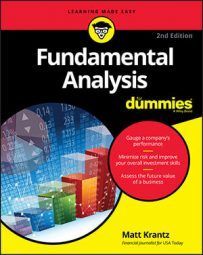##### Fundamental Analysis For DummiesIf you're going to attempt to pick individual stocks using fundamental analysis, you owe it to yourself to also learn how to track your performance. Every year, you should know how to calculate what your portfolio's return was and how much risk you took on in order to achieve that risk.

Being able to measure your portfolio's return and risk and compare it against the stock market is critical. If you're spending lots of time and money searching for stocks, and lagging the stock market, you might want to try a different strategy.

## How to measure your portfolio's return

Measuring your portfolio's return is pretty straightforward. Assuming you didn't add any fresh cash to the account during the year, simply subtract your portfolio's value at the end of the year from its value at the start of the year. Divide that difference by your portfolio's value at the beginning of the year and multiply by 100, and voila, that's your percentage gain for the year. This table provides a simple example of returns.

Here's a sample portfolio:

 Quarter ended Portfolio value December 2015 \$10,000 March 2016 \$10,050 June 2016 \$10,500 September 2016 \$10,501 December 2016 \$11,000
Using the table, you would measure your portfolio's return this way:

(\$11,000 – \$10,000) / \$10,000 = 0.10. Multiply by 100 to convert the answer into a percentage, or 10 percent. That is your annual return.

Be sure to compare the return of your portfolio to that of the Standard & Poor's 500 or another stock market index to find out if you're beating, or getting beaten by, the market.

## How to measure your portfolio's risk

Putting a number on your portfolio's risk is a little trickier than measuring your return. You'll need to figure out how volatile your returns are using a statistical measure called standard deviation.

To calculate your portfolio's standard deviation, you will first need to tabulate the percentage changes for each period. You can do this analysis based on any period of time, but since the preceding table is quarterly, you can practice with that. You just use the formula above and calculate the returns for each quarter. When you're done, you'll see a table that looks like what you see here.

Here's a sample portfolio of returns:

 Quarter ended Return March 2009 0.5% June 2009 4.5% September 2009 0.01% December 2009 4.8%
Lastly, you'll need to calculate the standard deviation. Enter the row and columns from your quarterly returns, like the ones in the preceding table, into Microsoft Excel. You can then use the `=stdev` function and let Excel do the number crunching for you.

If you have an HP 12C financial calculator, you can enter each return followed by the Sigma + key. When you're done entering all the returns, press the g key and the s key to see your portfolio's quarterly standard deviation. The answer you get is 2.55 percentage points.

You're almost done. You must now convert the quarterly standard deviation into an annualized number. You do that by multiplying the period's standard deviation by the square root of the number of periods in a year. But in this case, because you are measuring quarterly standard deviation, there are four quarters in a year. That means you take the square root of 4, which is 2. So to convert your quarterly standard deviation to an annualized figure, just multiply the 2.55 standard deviation by 2.

## Sizing up your portfolio's risk and return

Just knowing your portfolio's return and risk doesn't tell you much. It's important to compare those data with a benchmark, or a set of investments that you want to size yourself up against. The IFA Risk and Return Calculator lets you see the returns and standard deviations of several measures of stocks to see how you're doing.

Be careful of drawing too much from just one year. Don't assume that if you had one good year your luck will continue.